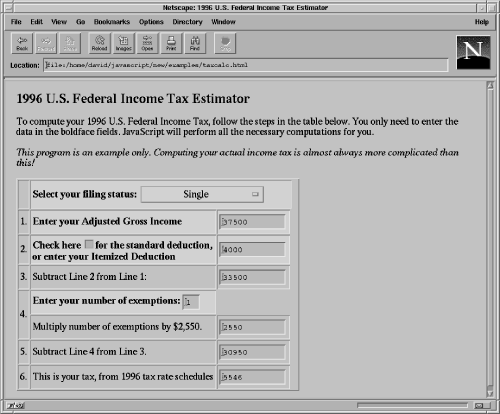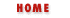#Chapter 1Introduction to JavaScript## 1.5 An Example: Calculating Your Taxes with JavaScript

Example 1.3 is a listing of a complete, non-trivial JavaScript program. The program calculates the estimated U.S. federal income tax you will have to pay for 1996. The program is displayed in Figure 1.3. As you can see, it consists of an HTML form displayed within an HTML table. To use it, you enter your filing status, adjusted gross income, and a couple of other pieces of data. Every time you enter data into the form, JavaScript recomputes all the fields and displays your estimated tax at the bottom.

 If you are not a U.S. resident, you won't have to pay, but you should study this example anyway!

#### Figure 1.3: A JavaScript tax estimatorThis example is a fairly complex one, but is worth taking the time to look over. You shouldn't expect to understand all the JavaScript code at this point, but studying the program will give you a good idea of what JavaScript programs look like, how event handlers work, and how JavaScript code can be integrated with HTML forms.

The beginning of the program defines "constructor functions" for two data types we'll use in the tax calculation. These new datatypes are TaxBracket and TaxSchedule. The next portion of the program creates and initializes an array of four TaxSchedule objects, each of which contains five TaxBracket objects. This is the data that the program will use to compute income tax.

Next comes the definition of a function named compute(). This is the function that computes the estimated tax you'll have to pay. It doesn't just perform the computation, however. It also reads the user's input from the form, and stores the result of the tax computation, along with intermediate results in the computation back into the form. The variable f in this function refers to the HTML form, and the various elements of the form are accessed by name. Thus, you'll see expressions like f.income.value to refer to the string that the user entered in the income field. The names for these fields will be assigned when the form is itself defined. Note that this compute() function both reads and writes the value of expressions like f.income.value and f.standard.checked--querying and setting the values displayed in the form. If you follow the comments, and refer occasionally to the reference section (Part III of this book), you may be able to follow the logic behind the tax computation.

After the definition of the compute() function, we reach the end of the JavaScript <SCRIPT>. The rest of the file consists of HTML, but this does not mean that JavaScript is not involved. After some brief instructions to the user, the HTML begins to define the form displayed by the program. The elements of the form are contained within an HTML table which makes things somewhat harder to figure out. Note, though, that every input element defined in the form has a NAME attribute, so that JavaScript can refer to it by name. And note that every input element has an event handler defined. These event handlers all call the compute() function defined earlier in the program. This means that whenever the user enters a value, all values in the form will be recomputed and redisplayed.

#### Example 1.3: Estimating Your Taxes with JavaScript

```<HEAD>
<TITLE>1996 U.S. Federal Income Tax Estimator</TITLE>
<SCRIPT>
// These functions define the data structures we'll use to store
// tax bracket and tax schedule data for computing taxes.
function TaxBracket(cutoff, percentage, base)
{
this.cutoff = cutoff;          // how much money to be in this bracket
this.percentage = percentage;  // what the tax is in this bracket
this.base = base;              // combined tax from all lower brackets
}
function TaxSchedule(b0, b1, b2, b3, b4)
{   // A tax schedule is just 5 brackets
this = b0; this = b1; this = b2; this = b3; this = b4;
}
// Taxes are computed using a tax schedule that depends on your filing status,
// so we create an array and store four different schedules in it.
var Schedules = new Object();  // create the array.
// Schedule X: Single
Schedules =  new TaxSchedule(new TaxBracket(263750, .396, 84020.5),
new TaxBracket(121300, .36, 32738.5), new TaxBracket(58150, .31, 13162),
new TaxBracket(24000, .28, 3600), new TaxBracket(0, .15, 0));
// Schedule Z: Head of Household
Schedules = new TaxSchedule(new TaxBracket(263750, .396, 81554),
new TaxBracket(134500, .36, 35024), new TaxBracket(83050, .31, 19074.5),
new TaxBracket(32150, .28, 4822.5), new TaxBracket(0, .15, 0));
// Schedule Y1: Married,  Filing Jointly
Schedules = new TaxSchedule(new TaxBracket(263750, .396, 79445),
new TaxBracket(147700, .36, 37667), new TaxBracket(96900, .31, 21919),
new TaxBracket(40100, .28, 6015), new TaxBracket(0, .15, 0));
// Schedule Y2: Married, Filing Separately
Schedules = new TaxSchedule(new TaxBracket(131875, .396, 39722.5),
new TaxBracket(73850, .36, 18833.5), new TaxBracket(48450, .31, 10959.5),
new TaxBracket(20050, .28, 3007.5),  new TaxBracket(0, .15, 0));
// The standard deduction allowed by tax law depends on filing status,
// so we've got to store this data in an array as well.
var StandardDeductions = new Object();
StandardDeductions = 4000; StandardDeductions = 5900;
StandardDeductions = 6700; StandardDeductions = 3350;
// This function computes the tax and updates all the elements in the form.
// It is triggered whenever anything changes, and makes sure that
// all elements of the form contain legal values and are consistent.
function compute()
{
var f = document.taxcalc;  // This is the form we'll we working with.
// get the filing status
var status = f.status.selectedIndex;
// line 1, adjusted gross income
var income = parseFloat(f.income.value);
if (isNaN(income)) { income = 0; f.income.value = "0"; }
f.income.value = Math.round(income);

// line 2, the standard or itemized deduction
var deduction;
if (f.standard.checked)
deduction = StandardDeductions[status];
else {
deduction = parseFloat(f.deduction.value);
if (isNaN(deduction)) deduction = 0;
if (deduction < StandardDeductions[status]) {
deduction = StandardDeductions[status];
f.standard.checked = true;
}
}
f.deduction.value = Math.round(deduction);
// Line 3: Subtract line 2 from line 1
var line3 = income - deduction;
if (line3 < 0) line3 = 0;
f.line3.value = line3;
// Line 4: exemptions
var num_exemptions = parseInt(f.num_exemptions.value);
if (isNaN(num_exemptions)) num_exemptions = 1;
f.num_exemptions.value = num_exemptions;
var exemption = num_exemptions * 2550;
f.exemption.value = exemption;
// Line 5: subtract Line 4 from Line 3.
var line5 = line3 - exemption;
if (line5 < 0) line5 = 0;
f.line5.value = line5;
// Line 6: tax from schedules.
// determine which tax schedule to use, based on filing status
var schedule = Schedules[status];
// determine which tax bracket to use within that schedule
for(var i = 0; i < 5; i++) if (line5 >= schedule[i].cutoff) break;
var bracket = schedule[i];
// then compute the tax based on that bracket
var tax = (line5 - bracket.cutoff) * bracket.percentage + bracket.base;
f.tax.value = Math.round(tax);
}
</SCRIPT>
<BODY>
<H1>1996 U.S. Federal Income Tax Estimator</H1>
To compute your 1996 U.S. Federal Income Tax, follow the steps in the
table below. You only need to enter the data in the boldface fields.
JavaScript will perform all the necessary computations for you.
<P>
<I>This program is an example only. Computing your actual income tax is
almost always more complicated than this!</I>
<!--
-- The code below is an HTML table inside of an HTML form. It gets tricky!
-- Notice the event handlers on all the form input elements.
-->
<FORM NAME="taxcalc">
<TR>                                        <!-- Filing status -->
<TD>&nbsp;</TD>
<TD COLSPAN=3 BGCOLOR="d0d0d0">
<SELECT NAME="status" onChange="compute()">
<OPTION VALUE=0 SELECTED>Single
<OPTION VALUE=2>Married, Filing Jointly
<OPTION VALUE=3>Married, Filing Separately
</SELECT>
</TD></TR>
<TR>                                        <!-- Line 1: Income -->
<TD>1.</TD>
<TD BGCOLOR="d0d0d0">
<INPUT TYPE=text NAME="income" SIZE=12 onChange="compute()">
</TD></TR>
<TR>                                        <!-- Line 2: Deduction -->
<TD>2.</TD>
<TD BGCOLOR="d0d0d0">
<B>Check here
<INPUT TYPE=checkbox NAME="standard" CHECKED onClick="compute()">
for the standard deduction,<BR>or enter your Itemized Deduction</B>
</TD>
<TD BGCOLOR="d0d0d0">
<INPUT TYPE=text NAME="deduction" SIZE=12
onChange="this.form.standard.checked = false; compute()">
</TD></TR>
<TR>                                        <!-- Line 3: subtraction -->
<TD>3.</TD>
<TD>Subtract Line 2 from Line 1:</TD>
<TD><INPUT TYPE=text NAME="line3" SIZE=12 onChange="compute()"></TD></TR>
<TR>                                        <!-- Line 4: Exemption -->
<TD ROWSPAN=2>4.</TD>
<TD BGCOLOR="d0d0d0">
<B>Enter your number of exemptions: </B>
<INPUT TYPE=text NAME="num_exemptions" SIZE=2 onChange="compute()">
</TD><TD></TD></TR>
<TR>                                        <!-- Line 4, continued -->
<TD>Multiply number of exemptions by \$2,550.</TD>
<TD><INPUT TYPE=text NAME="exemption" SIZE=12 onChange="compute()"></TD>
</TR>
<TR>                                        <!-- Line 5: subtraction -->
<TD>5.</TD>
<TD>Subtract Line 4 from Line 3.</TD>
<TD><INPUT TYPE=text NAME="line5" SIZE=12 onChange="compute()"></TD></TR>
<TR>                                        <!-- Line 6: Tax -->
<TD>6.</TD>
<TD>This is your tax, from 1996 tax rate schedules</TD>
<TD><INPUT TYPE=text NAME="tax" SIZE=12 onChange="compute()"></TD></TR>
</TABLE>
</FORM>
</BODY>
```What JavaScript Can't DoFlavors and Versions of JavaScript